How to Learn in 24 Hours?The Rapid Learning Movie

 Need Help? M-F: 9am-5pm(PST): Toll-Free: (877) RAPID-10 US Direct: (714) 692-2900 Int'l: 001-714-692-2900 24/7 Online Technical Support: The Rapid Support Center Secure Online Order:Need Proof? Testimonials by Our Users

 Rapid Learning Courses: MCAT in 24 Hours (2015-16) USMLE in 24 Hours (Boards) Chemistry in 24 Hours Biology in 24 Hours Physics in 24 Hours Mathematics in 24 Hours Psychology in 24 Hours SAT in 24 Hours ACT in 24 Hours AP in 24 Hours CLEP in 24 Hours DAT in 24 Hours (Dental) OAT in 24 Hours (Optometry) PCAT in 24 Hours (Pharmacy) Nursing Entrance Exams Certification in 24 Hours eBook - Survival Kits Audiobooks (MP3)

 Tell-A-Friend: Have friends taking science and math courses too? Tell them about our rapid learning system.Home »  Mathematics »  Intermediate Algebra

Basic Algebra Review

 Topic Review on "Title": Absolute Value: The absolute value of x defined as: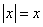if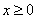and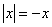if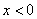. Properties of exponents: Multiplication of exponents rule is defined as: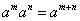Division of exponents rule is defined as: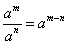The power rule of exponents is defined as: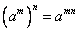Interval notation: Interval notation is another form of representing inequalities without having to use too much mathematical notation.

Rapid Study Kit for "Title":
 Flash Movie Flash Game Flash Card Core Concept Tutorial Problem Solving Drill Review Cheat Sheet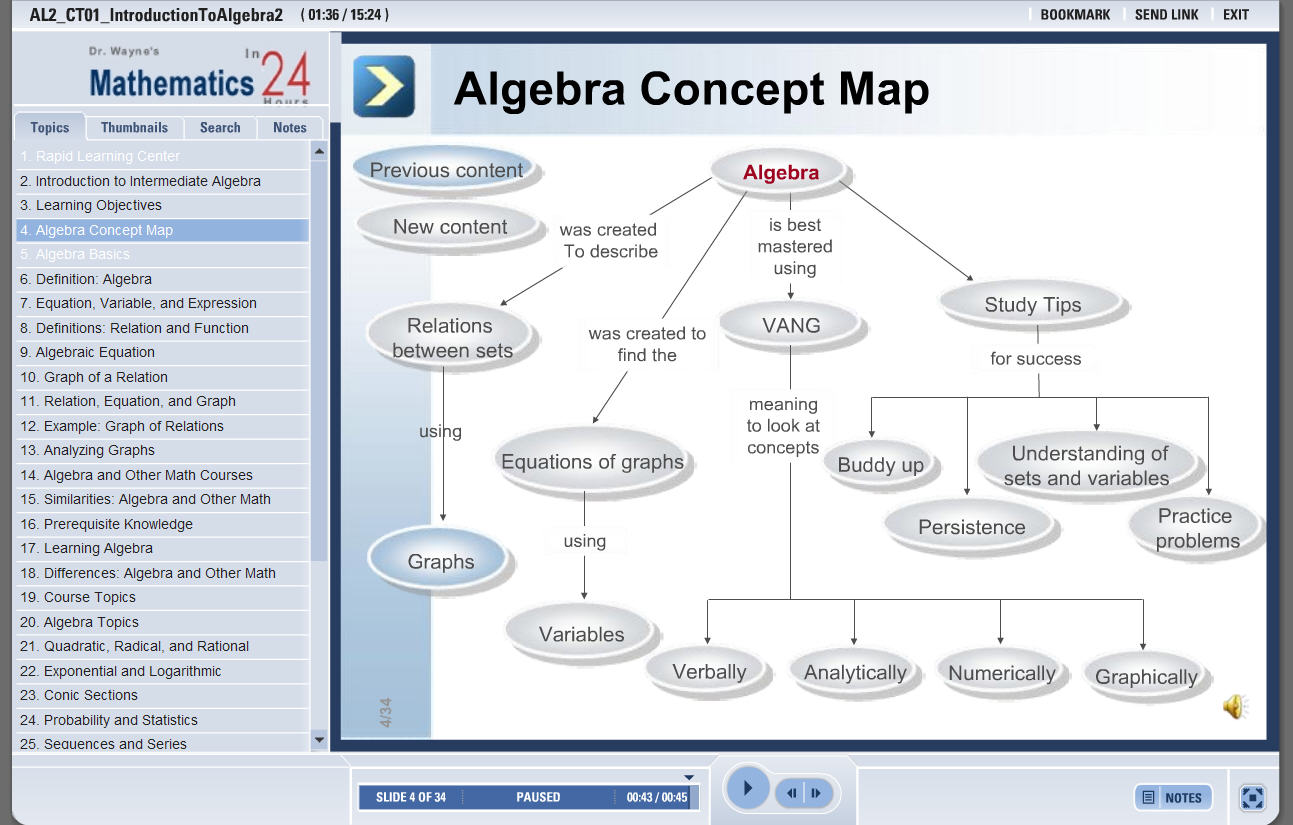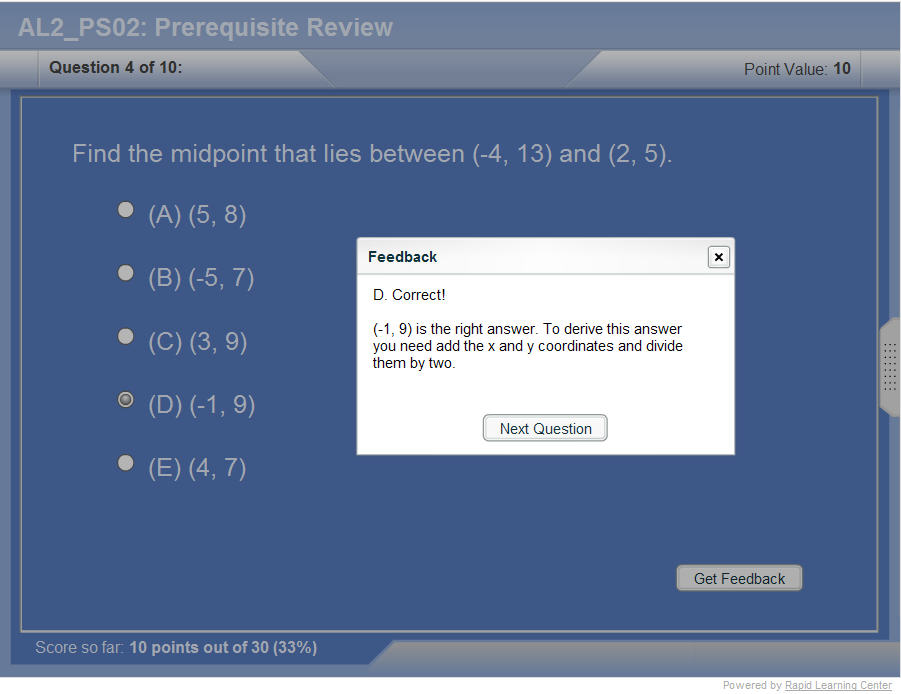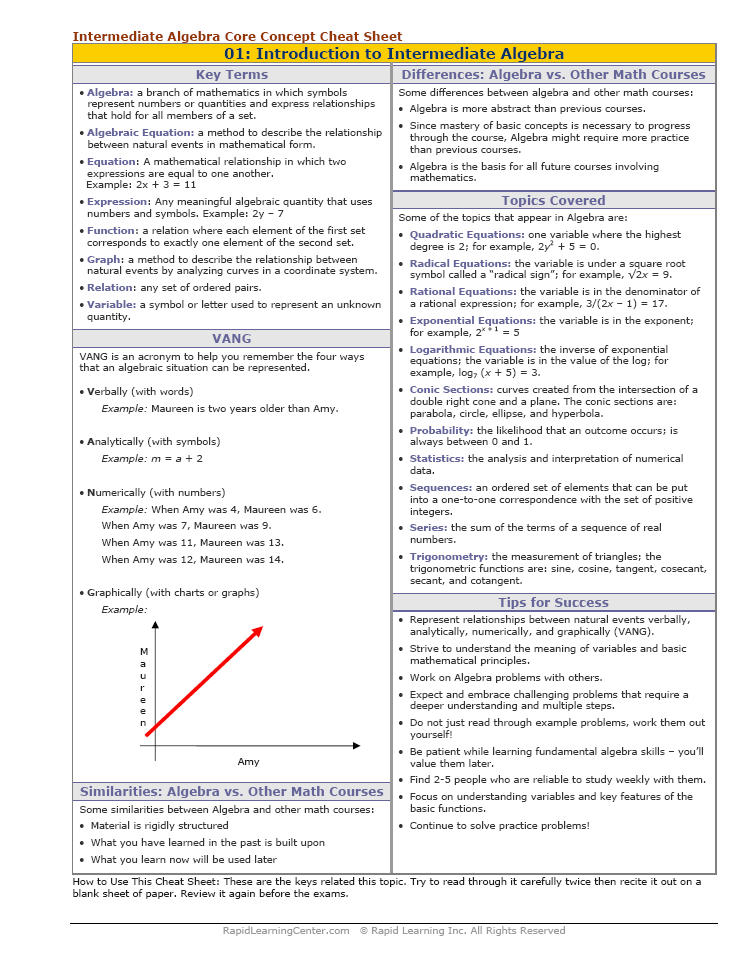"Title" Tutorial Summary : Intermediate Algebra prerequisites are covered in this tutorial. The use of real numbers and what operations are performed in example problems are introduced in this tutorial. A representation of inequalities using interval notation and the use of absolute values are presented in this tutorial. Important mathematics principles such as scientific notation and formulas are shown here to emphasize the importance of them in mathematics application problems. Scientific notation is commonly used in course such as chemistry and biochemistry to represent values.

 Tutorial Features: Specific Tutorial Features: • Step by step mathematical examples showing all details: Interval Notation, Absolute Values, Real Numbers and Their Operations, Algebraic Expressions, and Exponents Series Features: • Concept map showing inter-connections of new concepts in this tutorial and those previously introduced. • Definition slides introduce terms as they are needed. • Visual representation of concepts • Animated examples—worked out step by step • A concise summary is given at the conclusion of the tutorial.

 "Title" Topic List: Inequalities and their interval notation representationAbsolute Values and ExamplesThe operations of real numbersAlgebraic Expressions and ExamplesThe properties of exponentsScientific NotationFormulas and Examples

See all 24 lessons in Intermediate Algebra, including concept tutorials, problem drills and cheat sheets:
Teach Yourself Intermediate Algebra Visually in 24 Hours